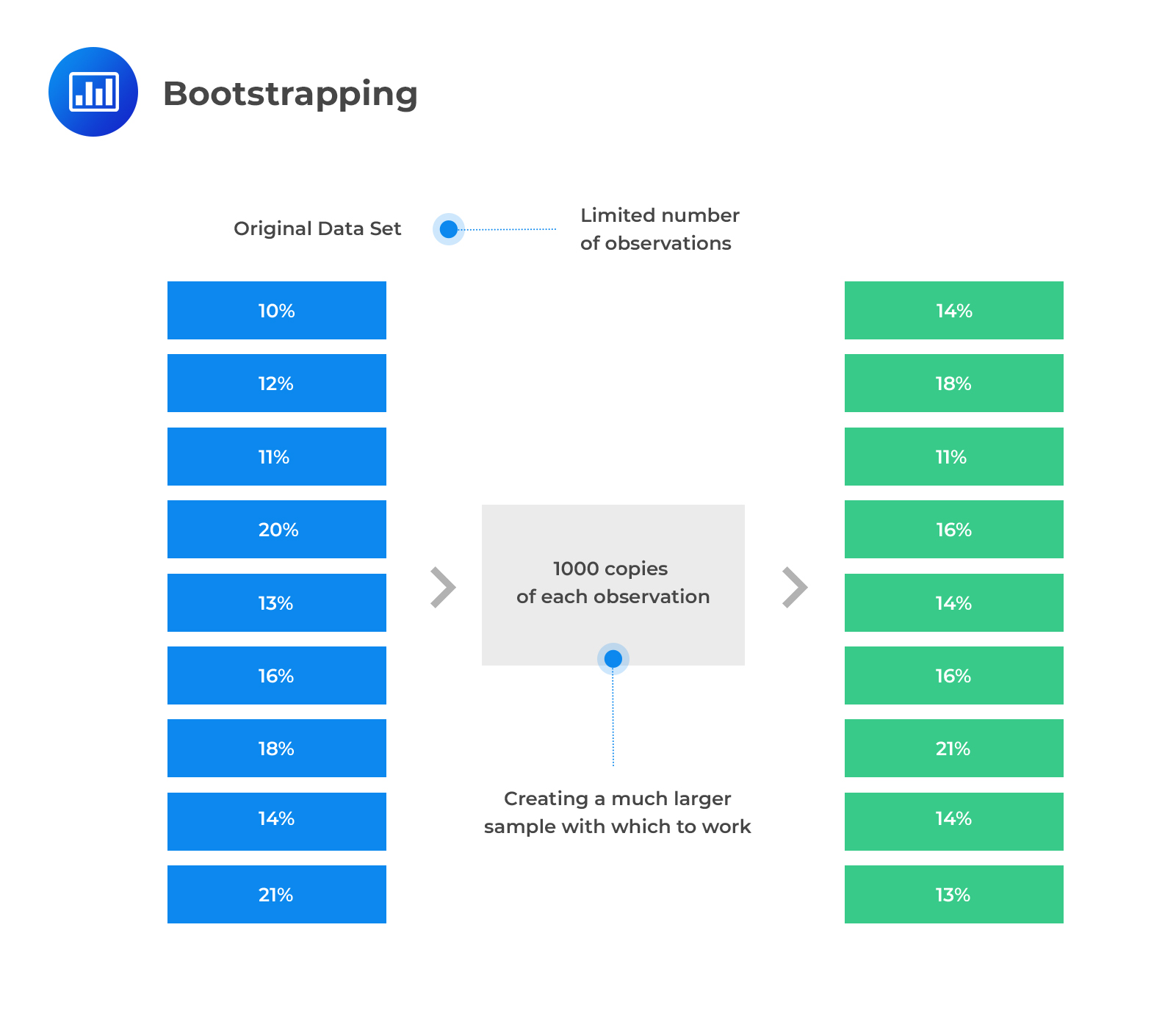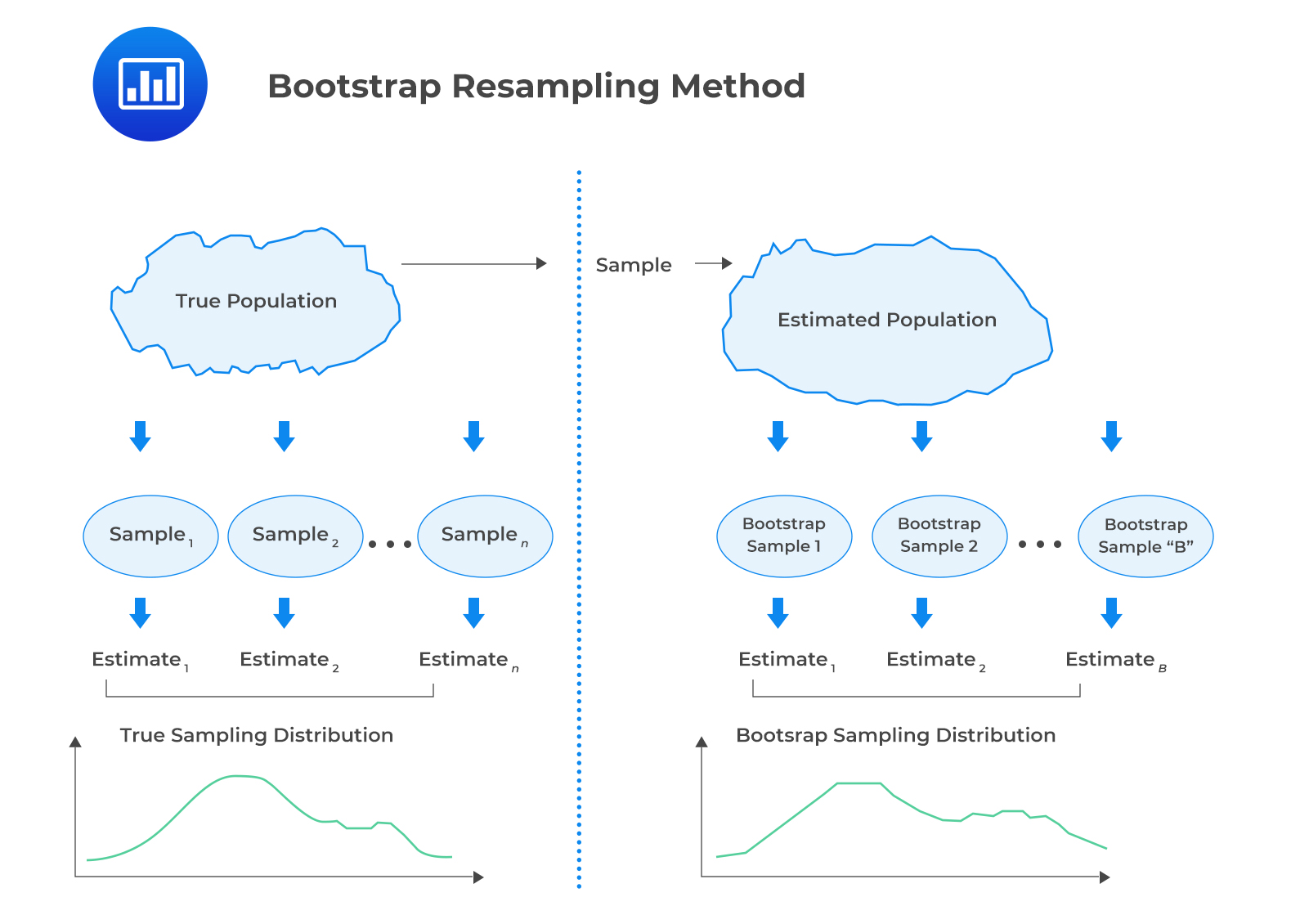Limited Time Offer: Save 10% on all 2022 Premium Study Packages with promo code: BLOG10# Resampling

Resampling refers to the act of repeatedly drawing samples from the original observed data sample for the statistical inference of population parameters. The following are the two commonly used methods of resampling: Bootstrap and jackknife.

## Bootstrap

In the bootstrap resampling method, a computer simulation is used to repeatedly draw random samples from the original sample. The resamples are then used to construct a sampling distribution.In the bootstrap method, the number of repeated samples to be drawn is at the discretion of the researcher. Furthermore, we can calculate the standard error of the mean. This is done by resampling and calculating the mean of each sample. The following formula is used to estimate the standard error.

$$s_{\bar{X}}=\sqrt{\frac{1}{B-1} \sum_{b=1}^{B}\left(\hat{\theta}_{b}-\bar{\theta}\right)^{2}}$$

Where:

$$s_{\bar{X}}$$ = Estimate of the standard error of the sample mean.

$$B$$ = Number of resamples drawn from the original sample.

$$\hat{\theta}_{b}$$ = Mean of a resample.

$$\bar{\theta}$$ = Mean across all the resample means.

The bootstrap resampling method can also be used in the estimation of the confidence intervals for the statistic. Alternatively, it can be used to find other population parameters.## Jackknife

Jackknife is a resampling method in which samples are selected by taking the original observed data sample and leaving out one observation at a time. As such, the samples are drawn without replacement. For a sample size of n, we need n repeated samples. This method can be used to reduce the bias of an estimator or to estimate the standard error and the confidence interval of an estimator.

## Question

Assume that you are studying the median height of 100 students in a university. You draw a sample of 1000 students and obtain 1000 median heights. The mean across all resample means is 5.8. The sum of squares of the differences between each sample mean and the mean across all resample means $$\left(\sum_{b=1}^{B}{\left(\hat{\theta}_{b}-\bar{\theta}\right)^{2}}\right)$$ is 2.3.

The estimate of the standard error of the sample mean is closest to:

1. 0.05.
2. 0.08.
3. 0.10.

Solution

The correct answer is A.

\begin{aligned} S_{\bar{X}}&=\sqrt{\frac{1}{B-1}} \sum_{b=1}^{B}\left(\hat{\theta}_{b}-\bar{\theta}\right) ^ 2 \\ &=\sqrt{\frac{1}{1000-1}}(2.3)=0.04798 \end{aligned}

Shop CFA® Exam Prep

Offered by AnalystPrepLevel I
Level II
Level III
All Three Levels
Featured Shop FRM® Exam PrepFRM Part I
FRM Part II
FRM Part I & Part II
Learn with Us

Subscribe to our newsletter and keep up with the latest and greatest tips for success
Shop Actuarial Exams PrepExam P (Probability)
Exam FM (Financial Mathematics)
Exams P & FM
Shop GMAT® Exam PrepComplete CourseSergio Torrico
2021-07-23
Excelente para el FRM 2 Escribo esta revisión en español para los hispanohablantes, soy de Bolivia, y utilicé AnalystPrep para dudas y consultas sobre mi preparación para el FRM nivel 2 (lo tomé una sola vez y aprobé muy bien), siempre tuve un soporte claro, directo y rápido, el material sale rápido cuando hay cambios en el temario de GARP, y los ejercicios y exámenes son muy útiles para practicar.diana
2021-07-17
So helpful. I have been using the videos to prepare for the CFA Level II exam. The videos signpost the reading contents, explain the concepts and provide additional context for specific concepts. The fun light-hearted analogies are also a welcome break to some very dry content. I usually watch the videos before going into more in-depth reading and they are a good way to avoid being overwhelmed by the sheer volume of content when you look at the readings.Kriti Dhawan
2021-07-16
A great curriculum provider. James sir explains the concept so well that rather than memorising it, you tend to intuitively understand and absorb them. Thank you ! Grateful I saw this at the right time for my CFA prep.nikhil kumar
2021-06-28
Very well explained and gives a great insight about topics in a very short time. Glad to have found Professor Forjan's lectures.Marwan
2021-06-22
Great support throughout the course by the team, did not feel neglectedBenjamin anonymous
2021-05-10
I loved using AnalystPrep for FRM. QBank is huge, videos are great. Would recommend to a friendDaniel Glyn
2021-03-24
I have finished my FRM1 thanks to AnalystPrep. And now using AnalystPrep for my FRM2 preparation. Professor Forjan is brilliant. He gives such good explanations and analogies. And more than anything makes learning fun. A big thank you to Analystprep and Professor Forjan. 5 stars all the way!michael walshe
2021-03-18
Professor James' videos are excellent for understanding the underlying theories behind financial engineering / financial analysis. The AnalystPrep videos were better than any of the others that I searched through on YouTube for providing a clear explanation of some concepts, such as Portfolio theory, CAPM, and Arbitrage Pricing theory. Watching these cleared up many of the unclarities I had in my head. Highly recommended.